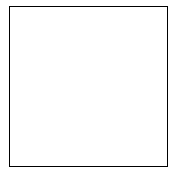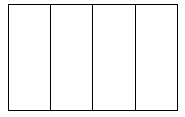# Fraction Equivalence and Ordering

## Objective

Recognize and generate equivalent fractions with smaller units using area models.

## Common Core Standards

### Core Standards

?

• 4.NF.A.1 — Explain why a fraction a/b is equivalent to a fraction (n × a)/(n × b) by using visual fraction models, with attention to how the number and size of the parts differ even though the two fractions themselves are the same size. Use this principle to recognize and generate equivalent fractions.

?

• 3.NF.A.3

• 4.OA.A.1

• 4.OA.A.2

## Criteria for Success

?

1. Understand that equivalent fractions are fractions that refer to the same whole and are the same size.
2. Generate equivalent fractions with smaller units using visual models, namely area models (MP.5).
3. Generate equivalent fractions with smaller units when given either the number of units (the numerator) or the size of the unit (the denominator) in the equivalent fraction.
4. Determine whether two fractions are equivalent using visual models and explain why the models demonstrate their equivalence (MP.3).

## Tips for Teachers

?

As a supplement to the Problem Set, students can create equivalent fractions using the following interactive Equivalent Fractions made available by NCTM Illuminations.

#### Fishtank Plus

• Problem Set
• Student Handout Editor
• Vocabulary Package

?

### Problem 1

1. A garden is divided into rows of various vegetables. Three out of four rows are tomatoes. Partition and shade the square below to show how much of the garden is tomatoes.1. The garden is going to be shared with family and friends so that each person’s share of the harvest is three-fourths tomatoes. How would you partition the garden if it’s shared by two people? Show on the model above.
2. What fraction of the newly divided garden is corn when it is shared by two people? Write an equation to show this relationship.
3. Go through the same process for the garden but now splitting it evenly for three and five people on the models below.#### References

John A. Van de Walle Teaching Student-Centered Mathematics: Developmentally Appropriate Instruction for Grades 3-5 (Volume II)Activity 12.16

Van de Walle, John A. Teaching Student-Centered Mathematics: Developmentally Appropriate Instruction for Grades 3-5 (Volume II). Pearson, 2nd edition, 2013.

Modified by The Match Foundation, Inc.

### Problem 2

Find two fractions that are equivalent to each of the following:

a.   ${{1\over8}}$

b.   ${{5\over12}}$

c.   ${{4\over3}}$

### Problem 3

Solve for the missing value.

a.     ${{2\over5}={10\over \square}}$

b.     ${{5\over4}={\square\over16}}$

## Problem Set & Homework

#### Discussion of Problem Set

• Discuss students’ work on the NCTM Illuminations Interactive. You could make connections between the area model and the number line. You could also demonstrate how the area model can be partitioned in just one dimension for some fractions (e.g., to find a fraction equivalent to ${{2\over3}}$, students can just create a model with 6 rows, rather than 3 rows and 2 columns (or vice versa), which nicely connects to students’ work in Lesson 1. Further, you could discuss the use of the circle area model since students also saw circular models on the Problem Set.
• How is the model in #2 different from the ones we’ve already seen? How is it similar?
• How did you determine how many pieces of cake were left in #3? How did the model help you?
• Look at #5. How many slices of pizza did he have left? How did you find that? If each friend ate ${{1\over4}}$ of the pizza, how many slices did they eat?
• How did you decide how many squares to shade in #6? What fraction is represented in the model? What about #7?

?

The model below is made up of equal-sized pieces.Explain how you can use the model to show ${{{{9\over12}}}}$. Then write a fraction that is equivalent to ${{{{9\over12}}}}$.

?